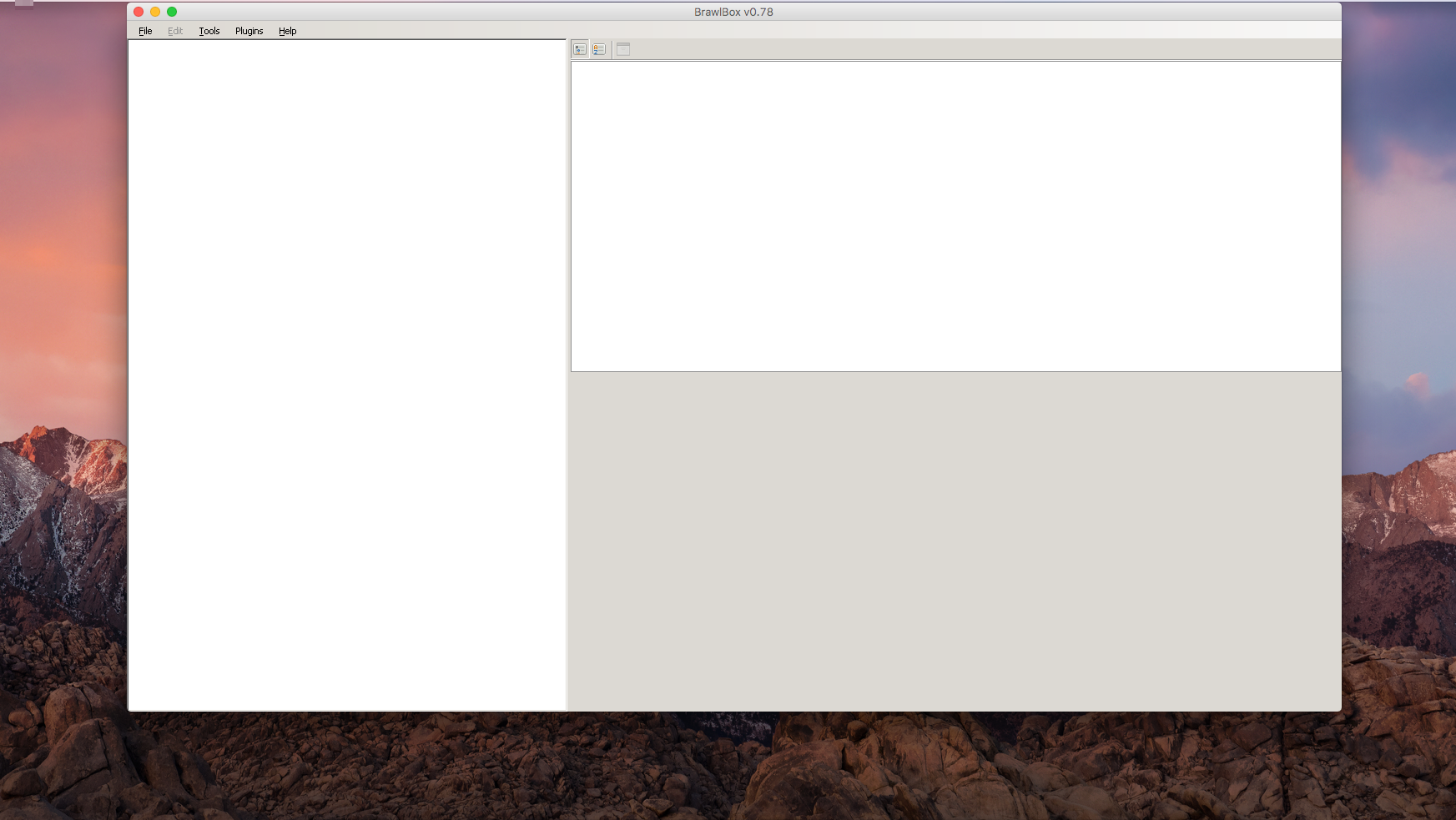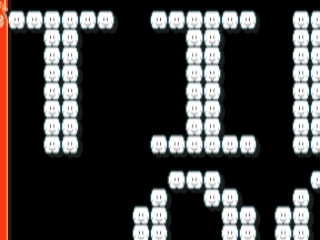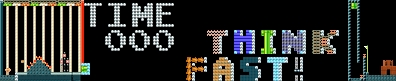ModMakerIdk how im second most active I'm now 99.9^100% dead Normal user Since: 07-23-17 (I am not a windows user so this MAY not work) Step 1: Install Super Mario ReMaker here Step 2: Go to your Downloads and extract the .Zip file. Step 3: When you open the file you should see a file called "SuperMarioReMaker.exe" Step 4: Run the .exe file (If you don't know how to run a .exe file on windows click here) Step 5: Enjoy!_________________________Mr.M0d Posted on 08-19-17, 04:51 pm in windows user How to Run Super Mario ReMaker on Windows blargModMakerIdk how im second most active I'm now 99.9^100% dead Normal user Since: 07-23-17 Simple, but effective! Last year, someone did some research into level stats. I think it's when Mario Maker had around 10 million levels. It was something like 3 million NSMBU Overworlds and only 10k SMB3 Underwaters. I just find it hard to decorate properly, personally. I did make an autoscrolling boot level with it, though. Still, nice fix! Posted by MayroSMM Good job with that!_________________________Mr.M0d Posted on 08-20-17, 02:01 pm in 3ds SMB3 Underwater Deluxe blargModMakerIdk how im second most active I'm now 99.9^100% dead Normal user Since: 07-23-17 Can someone do me a favor and extract the .rar and send a link of the file to me? _________________________Mr.M0d Posted on 08-20-17, 02:02 pm in 3dssmwwii uswitch Volcano Theme blargModMakerIdk how im second most active I'm now 99.9^100% dead Normal user Since: 07-23-17 How do you do that? Can I even use it because of my Mac?_________________________Mr.M0d Posted on 08-20-17, 02:04 pm in remaker Sans' Legend of a Bad Time blargModMakerIdk how im second most active I'm now 99.9^100% dead Normal user Since: 07-23-17 All I'm getting is this What the heck do I do BTW a Mac app data is aka Application Support so I put this file in there? [misc] time="400.000000" yarrow="912.000000" xarrow="32.000000" type="8.000000" width="2400.000000" [course] 5292="864.000000" 5291="2304.000000" 5290="141.000000" 5282="832.000000" 5281="832.000000" 5280="33.000000" 5272="832.000000" 5271="1392.000000" 5270="33.000000" 5262="800.000000" 5261="1760.000000" 5260="33.000000" 5252="800.000000" 5251="1856.000000" 5250="33.000000" 5242="912.000000" 5241="1232.000000" 5240="75.000000" 524C="3.000000" 5232="912.000000" 5231="224.000000" 5230="20.000000" 5222="896.000000" 5221="432.000000" 5220="18.000000" 5212="880.000000" 5211="448.000000" 5210="18.000000" 5202="864.000000" 5201="464.000000" 5200="18.000000" 5192="928.000000" 5191="1968.000000" 5190="19.000000" 5182="928.000000" 5181="2000.000000" 5180="18.000000" 5172="928.000000" 5171="2016.000000" 5170="18.000000" 5162="928.000000" 5161="2032.000000" 5160="18.000000" 5152="896.000000" 5151="48.000000" 5150="75.000000" 515C="3.000000" 5142="896.000000" 5141="704.000000" 5140="63.000000" 5132="896.000000" 5131="720.000000" 5130="63.000000" 5122="896.000000" 5121="736.000000" 5120="63.000000" 5112="896.000000" 5111="752.000000" 5110="63.000000" 5102="896.000000" 5101="768.000000" 5100="63.000000" 5092="896.000000" 5091="784.000000" 5090="63.000000" 5082="896.000000" 5081="800.000000" 5080="63.000000" 5072="896.000000" 5071="832.000000" 5070="63.000000" 5062="896.000000" 5061="848.000000" 5060="63.000000" 5052="896.000000" 5051="864.000000" 5050="63.000000" 5042="896.000000" 5041="880.000000" 5040="63.000000" 5032="896.000000" 5031="896.000000" 5030="63.000000" 5022="896.000000" 5021="912.000000" 5020="63.000000" 5012="896.000000" 5011="928.000000" 5010="63.000000" 5002="896.000000" 5001="944.000000" 5000="63.000000" 4992="928.000000" 4991="1248.000000" 4990="6.000000" 4982="928.000000" 4981="1232.000000" 4980="6.000000" 4972="928.000000" 4971="1216.000000" 4970="6.000000" 4962="912.000000" 4961="1216.000000" 4960="6.000000" 4952="912.000000" 4951="1248.000000" 4950="6.000000" 4942="864.000000" 4941="1424.000000" 4940="5.000000" 4932="864.000000" 4931="1408.000000" 4930="5.000000" 4922="864.000000" 4921="1392.000000" 4920="5.000000" 4912="864.000000" 4911="1376.000000" 4910="5.000000" 4902="864.000000" 4901="1360.000000" 4900="5.000000" 4892="864.000000" 4891="1344.000000" 4890="5.000000" 4882="880.000000" 4881="1344.000000" 4880="5.000000" 4872="880.000000" 4871="1360.000000" 4870="5.000000" 4862="880.000000" 4861="1376.000000" 4860="5.000000" 4852="880.000000" 4851="1392.000000" 4850="5.000000" 4842="880.000000" 4841="1408.000000" 4840="5.000000" 4832="880.000000" 4831="1424.000000" 4830="5.000000" 4822="896.000000" 4821="1424.000000" 4820="5.000000" 4812="896.000000" 4811="1408.000000" 4810="5.000000" 4802="896.000000" 4801="1392.000000" 4800="5.000000" 4792="896.000000" 4791="1376.000000" 4790="5.000000" 4782="896.000000" 4781="1360.000000" 4780="5.000000" 4772="896.000000" 4771="1344.000000" 4770="5.000000" 4762="912.000000" 4761="1344.000000" 4760="5.000000" 4752="912.000000" 4751="1360.000000" 4750="5.000000" 4742="912.000000" 4741="1376.000000" 4740="5.000000" 4732="912.000000" 4731="1392.000000" 4730="5.000000" 4722="912.000000" 4721="1408.000000" 4720="5.000000" 4712="912.000000" 4711="1424.000000" 4710="5.000000" 4702="928.000000" 4701="1424.000000" 4700="5.000000" 4692="928.000000" 4691="1408.000000" 4690="5.000000" 4682="928.000000" 4681="1392.000000" 4680="5.000000" 4672="928.000000" 4671="1376.000000" 4670="5.000000" 4662="944.000000" 4661="1344.000000" 4660="5.000000" 4652="928.000000" 4651="1344.000000" 4650="5.000000" 4642="928.000000" 4641="1360.000000" 4640="5.000000" 4632="944.000000" 4631="1360.000000" 4630="5.000000" 4622="944.000000" 4621="1376.000000" 4620="5.000000" 4612="944.000000" 4611="1392.000000" 4610="5.000000" 4602="944.000000" 4601="1408.000000" 4600="5.000000" 4592="944.000000" 4591="1424.000000" 4590="5.000000" 4582="944.000000" 4581="1440.000000" 4580="5.000000" 4572="928.000000" 4571="1440.000000" 4570="5.000000" 4562="912.000000" 4561="1440.000000" 4560="5.000000" 4552="896.000000" 4551="1440.000000" 4550="5.000000" 4542="880.000000" 4541="1440.000000" 4540="5.000000" 4532="864.000000" 4531="1440.000000" 4530="5.000000" 4522="848.000000" 4521="1440.000000" 4520="5.000000" 4512="848.000000" 4511="1424.000000" 4510="5.000000" 4502="848.000000" 4501="1408.000000" 4500="5.000000" 4492="848.000000" 4491="1392.000000" 4490="5.000000" 4482="848.000000" 4481="1376.000000" 4480="5.000000" 4472="848.000000" 4471="1360.000000" 4470="5.000000" 4462="848.000000" 4461="1344.000000" 4460="5.000000" 4452="848.000000" 4451="1328.000000" 4450="5.000000" 4442="864.000000" 4441="1328.000000" 4440="5.000000" 4432="880.000000" 4431="1328.000000" 4430="5.000000" 4422="896.000000" 4421="1328.000000" 4420="5.000000" 4412="912.000000" 4411="1328.000000" 4410="5.000000" 4402="928.000000" 4401="1328.000000" 4400="5.000000" 4392="944.000000" 4391="1328.000000" 4390="5.000000" 4382="832.000000" 4381="1264.000000" 4380="5.000000" 4372="848.000000" 4371="1264.000000" 4370="5.000000" 4362="816.000000" 4361="1264.000000" 4360="5.000000" 4352="800.000000" 4351="1264.000000" 4350="5.000000" 4342="784.000000" 4341="1264.000000" 4340="5.000000" 4332="768.000000" 4331="1264.000000" 4330="5.000000" 4322="752.000000" 4321="1264.000000" 4320="5.000000" 4312="736.000000" 4311="1264.000000" 4310="5.000000" 4302="720.000000" 4301="1264.000000" 4300="5.000000" 4292="704.000000" 4291="1264.000000" 4290="5.000000" 4282="688.000000" 4281="1264.000000" 4280="5.000000" 4272="672.000000" 4271="1264.000000" 4270="5.000000" 4262="656.000000" 4261="1264.000000" 4260="5.000000" 4252="640.000000" 4251="1264.000000" 4250="5.000000" 4242="624.000000" 4241="1264.000000" 4240="5.000000" 4232="608.000000" 4231="1264.000000" 4230="5.000000" 4222="592.000000" 4221="1264.000000" 4220="5.000000" 4212="576.000000" 4211="1264.000000" 4210="5.000000" 4202="944.000000" 4201="1312.000000" 4200="5.000000" 4192="912.000000" 4191="1312.000000" 4190="5.000000" 4182="928.000000" 4181="1312.000000" 4180="5.000000" 4172="944.000000" 4171="1296.000000" 4170="5.000000" 4162="928.000000" 4161="1296.000000" 4160="5.000000" 4152="912.000000" 4151="1296.000000" 4150="5.000000" 4142="944.000000" 4141="1280.000000" 4140="5.000000" 4132="928.000000" 4131="1280.000000" 4130="5.000000" 4122="912.000000" 4121="1280.000000" 4120="5.000000" 4112="944.000000" 4111="1264.000000" 4110="5.000000" 4102="928.000000" 4101="1264.000000" 4100="5.000000" 4092="912.000000" 4091="1264.000000" 4090="5.000000" 4082="944.000000" 4081="1248.000000" 4080="5.000000" 4072="944.000000" 4071="1232.000000" 4070="5.000000" 4062="944.000000" 4061="1216.000000" 4060="5.000000" 4052="912.000000" 4051="1200.000000" 4050="5.000000" 4042="912.000000" 4041="1184.000000" 4040="5.000000" 4032="928.000000" 4031="1184.000000" 4030="5.000000" 4022="928.000000" 4021="1200.000000" 4020="5.000000" 4012="944.000000" 4011="1200.000000" 4010="5.000000" 4002="944.000000" 4001="1184.000000" 4000="5.000000" 3992="944.000000" 3991="1168.000000" 3990="5.000000" 3982="928.000000" 3981="1168.000000" 3980="5.000000" 3972="912.000000" 3971="1168.000000" 3970="5.000000" 3962="944.000000" 3961="1088.000000" 3960="5.000000" 3952="928.000000" 3951="1088.000000" 3950="5.000000" 3942="912.000000" 3941="1088.000000" 3940="5.000000" 3932="912.000000" 3931="1072.000000" 3930="5.000000" 3922="928.000000" 3921="1072.000000" 3920="5.000000" 3912="944.000000" 3911="1072.000000" 3910="5.000000" 3902="912.000000" 3901="1024.000000" 3900="5.000000" 3892="928.000000" 3891="1024.000000" 3890="5.000000" 3882="944.000000" 3881="1024.000000" 3880="5.000000" 3872="864.000000" 3871="880.000000" 3870="5.000000" 3862="864.000000" 3861="864.000000" 3860="5.000000" 3852="864.000000" 3851="848.000000" 3850="5.000000" 3842="864.000000" 3841="832.000000" 3840="5.000000" 3832="864.000000" 3831="816.000000" 3830="5.000000" 3822="864.000000" 3821="800.000000" 3820="5.000000" 3812="864.000000" 3811="784.000000" 3810="5.000000" 3802="864.000000" 3801="768.000000" 3800="5.000000" 3792="880.000000" 3791="880.000000" 3790="5.000000" 3782="880.000000" 3781="864.000000" 3780="5.000000" 3772="880.000000" 3771="848.000000" 3770="5.000000" 3762="880.000000" 3761="832.000000" 3760="5.000000" 3752="880.000000" 3751="816.000000" 3750="5.000000" 3742="880.000000" 3741="800.000000" 3740="5.000000" 3732="880.000000" 3731="784.000000" 3730="5.000000" 3722="880.000000" 3721="768.000000" 3720="5.000000" 3712="912.000000" 3711="880.000000" 3710="5.000000" 3702="912.000000" 3701="864.000000" 3700="5.000000" 3692="912.000000" 3691="848.000000" 3690="5.000000" 3682="912.000000" 3681="832.000000" 3680="5.000000" 3672="912.000000" 3671="816.000000" 3670="5.000000" 3662="912.000000" 3661="800.000000" 3660="5.000000" 3652="912.000000" 3651="784.000000" 3650="5.000000" 3642="912.000000" 3641="768.000000" 3640="5.000000" 3632="928.000000" 3631="880.000000" 3630="5.000000" 3622="928.000000" 3621="864.000000" 3620="5.000000" 3612="928.000000" 3611="848.000000" 3610="5.000000" 3602="928.000000" 3601="832.000000" 3600="5.000000" 3592="928.000000" 3591="816.000000" 3590="5.000000" 3582="928.000000" 3581="800.000000" 3580="5.000000" 3572="928.000000" 3571="784.000000" 3570="5.000000" 3562="928.000000" 3561="768.000000" 3560="5.000000" 3552="944.000000" 3551="880.000000" 3550="5.000000" 3542="944.000000" 3541="864.000000" 3540="5.000000" 3532="944.000000" 3531="848.000000" 3530="5.000000" 3522="944.000000" 3521="832.000000" 3520="5.000000" 3512="944.000000" 3511="816.000000" 3510="5.000000" 3502="944.000000" 3501="800.000000" 3500="5.000000" 3492="944.000000" 3491="784.000000" 3490="5.000000" 3482="944.000000" 3481="768.000000" 3480="5.000000" 3472="944.000000" 3471="960.000000" 3470="5.000000" 3462="928.000000" 3461="960.000000" 3460="5.000000" 3452="912.000000" 3451="960.000000" 3450="5.000000" 3442="944.000000" 3441="944.000000" 3440="5.000000" 3432="928.000000" 3431="944.000000" 3430="5.000000" 3422="912.000000" 3421="944.000000" 3420="5.000000" 3412="944.000000" 3411="928.000000" 3410="5.000000" 3402="928.000000" 3401="928.000000" 3400="5.000000" 3392="912.000000" 3391="928.000000" 3390="5.000000" 3382="880.000000" 3381="928.000000" 3380="5.000000" 3372="912.000000" 3371="896.000000" 3370="5.000000" 3362="880.000000" 3361="912.000000" 3360="5.000000" 3352="912.000000" 3351="912.000000" 3350="5.000000" 3342="928.000000" 3341="912.000000" 3340="5.000000" 3332="944.000000" 3331="912.000000" 3330="5.000000" 3322="944.000000" 3321="896.000000" 3320="5.000000" 3312="928.000000" 3311="896.000000" 3310="5.000000" 3302="880.000000" 3301="896.000000" 3300="5.000000" 3292="864.000000" 3291="896.000000" 3290="5.000000" 3282="864.000000" 3281="912.000000" 3280="5.000000" 3272="848.000000" 3271="896.000000" 3270="5.000000" 3262="848.000000" 3261="880.000000" 3260="5.000000" 3252="848.000000" 3251="864.000000" 3250="5.000000" 3242="848.000000" 3241="848.000000" 3240="5.000000" 3232="848.000000" 3231="832.000000" 3230="5.000000" 3222="848.000000" 3221="816.000000" 3220="5.000000" 3212="848.000000" 3211="800.000000" 3210="5.000000" 3202="848.000000" 3201="784.000000" 3200="5.000000" 3192="848.000000" 3191="768.000000" 3190="5.000000" 3182="848.000000" 3181="752.000000" 3180="5.000000" 3172="864.000000" 3171="752.000000" 3170="5.000000" 3162="880.000000" 3161="752.000000" 3160="5.000000" 3152="912.000000" 3151="752.000000" 3150="5.000000" 3142="928.000000" 3141="752.000000" 3140="5.000000" 3132="944.000000" 3131="752.000000" 3130="5.000000" 3122="864.000000" 3121="736.000000" 3120="5.000000" 3112="880.000000" 3111="736.000000" 3110="5.000000" 3102="912.000000" 3101="736.000000" 3100="5.000000" 3092="928.000000" 3091="736.000000" 3090="5.000000" 3082="944.000000" 3081="736.000000" 3080="5.000000" 3072="880.000000" 3071="720.000000" 3070="5.000000" 3062="912.000000" 3061="720.000000" 3060="5.000000" 3052="928.000000" 3051="720.000000" 3050="5.000000" 3042="944.000000" 3041="720.000000" 3040="5.000000" 3032="912.000000" 3031="704.000000" 3030="5.000000" 3022="928.000000" 3021="704.000000" 3020="5.000000" 3012="944.000000" 3011="704.000000" 3010="5.000000" 3002="912.000000" 3001="688.000000" 3000="5.000000" 2992="928.000000" 2991="688.000000" 2990="5.000000" 2982="944.000000" 2981="688.000000" 2980="5.000000" 2972="864.000000" 2971="576.000000" 2970="5.000000" 2962="864.000000" 2961="560.000000" 2960="5.000000" 2952="864.000000" 2951="544.000000" 2950="5.000000" 2942="864.000000" 2941="528.000000" 2940="5.000000" 2932="864.000000" 2931="512.000000" 2930="5.000000" 2922="864.000000" 2921="496.000000" 2920="5.000000" 2912="880.000000" 2911="480.000000" 2910="5.000000" 2902="880.000000" 2901="496.000000" 2900="5.000000" 2892="880.000000" 2891="512.000000" 2890="5.000000" 2882="880.000000" 2881="528.000000" 2880="5.000000" 2872="880.000000" 2871="544.000000" 2870="5.000000" 2862="880.000000" 2861="560.000000" 2860="5.000000" 2852="880.000000" 2851="576.000000" 2850="5.000000" 2842="880.000000" 2841="592.000000" 2840="5.000000" 2832="896.000000" 2831="608.000000" 2830="5.000000" 2822="896.000000" 2821="592.000000" 2820="5.000000" 2812="896.000000" 2811="576.000000" 2810="5.000000" 2802="896.000000" 2801="560.000000" 2800="5.000000" 2792="896.000000" 2791="544.000000" 2790="5.000000" 2782="896.000000" 2781="528.000000" 2780="5.000000" 2772="896.000000" 2771="512.000000" 2770="5.000000" 2762="896.000000" 2761="496.000000" 2760="5.000000" 2752="896.000000" 2751="480.000000" 2750="5.000000" 2742="896.000000" 2741="464.000000" 2740="5.000000" 2732="912.000000" 2731="448.000000" 2730="5.000000" 2722="912.000000" 2721="464.000000" 2720="5.000000" 2712="912.000000" 2711="480.000000" 2710="5.000000" 2702="912.000000" 2701="496.000000" 2700="5.000000" 2692="912.000000" 2691="512.000000" 2690="5.000000" 2682="912.000000" 2681="528.000000" 2680="5.000000" 2672="912.000000" 2671="544.000000" 2670="5.000000" 2662="912.000000" 2661="560.000000" 2660="5.000000" 2652="912.000000" 2651="576.000000" 2650="5.000000" 2642="912.000000" 2641="592.000000" 2640="5.000000" 2632="912.000000" 2631="608.000000" 2630="5.000000" 2622="912.000000" 2621="624.000000" 2620="5.000000" 2612="928.000000" 2611="624.000000" 2610="5.000000" 2602="928.000000" 2601="608.000000" 2600="5.000000" 2592="928.000000" 2591="592.000000" 2590="5.000000" 2582="928.000000" 2581="576.000000" 2580="5.000000" 2572="928.000000" 2571="560.000000" 2570="5.000000" 2562="928.000000" 2561="496.000000" 2560="5.000000" 2552="928.000000" 2551="480.000000" 2550="5.000000" 2542="928.000000" 2541="464.000000" 2540="5.000000" 2532="928.000000" 2531="432.000000" 2530="5.000000" 2522="928.000000" 2521="448.000000" 2520="5.000000" 2512="944.000000" 2511="528.000000" 2510="5.000000" 2502="944.000000" 2501="432.000000" 2500="5.000000" 2492="944.000000" 2491="448.000000" 2490="5.000000" 2482="944.000000" 2481="464.000000" 2480="5.000000" 2472="944.000000" 2471="480.000000" 2470="5.000000" 2462="944.000000" 2461="496.000000" 2460="5.000000" 2452="944.000000" 2451="512.000000" 2450="5.000000" 2442="928.000000" 2441="512.000000" 2440="5.000000" 2432="928.000000" 2431="528.000000" 2430="5.000000" 2422="928.000000" 2421="544.000000" 2420="5.000000" 2412="944.000000" 2411="544.000000" 2410="5.000000" 2402="944.000000" 2401="560.000000" 2400="5.000000" 2392="944.000000" 2391="576.000000" 2390="5.000000" 2382="944.000000" 2381="592.000000" 2380="5.000000" 2372="944.000000" 2371="608.000000" 2370="5.000000" 2362="944.000000" 2361="624.000000" 2360="5.000000" 2352="944.000000" 2351="640.000000" 2350="5.000000" 2342="928.000000" 2341="640.000000" 2340="5.000000" 2332="912.000000" 2331="640.000000" 2330="5.000000" 2322="896.000000" 2321="624.000000" 2320="5.000000" 2312="880.000000" 2311="608.000000" 2310="5.000000" 2302="864.000000" 2301="592.000000" 2300="5.000000" 2292="848.000000" 2291="576.000000" 2290="5.000000" 2282="848.000000" 2281="560.000000" 2280="5.000000" 2272="848.000000" 2271="528.000000" 2270="5.000000" 2262="848.000000" 2261="512.000000" 2260="5.000000" 2252="848.000000" 2251="496.000000" 2250="5.000000" 2242="864.000000" 2241="480.000000" 2240="5.000000" 2232="880.000000" 2231="464.000000" 2230="5.000000" 2222="896.000000" 2221="448.000000" 2220="5.000000" 2212="912.000000" 2211="432.000000" 2210="5.000000" 2202="928.000000" 2201="416.000000" 2200="5.000000" 2192="944.000000" 2191="416.000000" 2190="5.000000" 2182="928.000000" 2181="368.000000" 2180="5.000000" 2172="928.000000" 2171="0.000000" 2170="5.000000" 2162="928.000000" 2161="16.000000" 2160="5.000000" 2152="928.000000" 2151="32.000000" 2150="5.000000" 2142="928.000000" 2141="48.000000" 2140="5.000000" 2132="928.000000" 2131="112.000000" 2130="5.000000" 2122="928.000000" 2121="128.000000" 2120="5.000000" 2112="928.000000" 2111="144.000000" 2110="5.000000" 2102="928.000000" 2101="160.000000" 2100="5.000000" 2092="944.000000" 2091="160.000000" 2090="5.000000" 2082="944.000000" 2081="144.000000" 2080="5.000000" 2072="944.000000" 2071="128.000000" 2070="5.000000" 2062="944.000000" 2061="112.000000" 2060="5.000000" 2052="944.000000" 2051="96.000000" 2050="5.000000" 2042="944.000000" 2041="80.000000" 2040="5.000000" 2032="944.000000" 2031="64.000000" 2030="5.000000" 2022="944.000000" 2021="48.000000" 2020="5.000000" 2012="944.000000" 2011="32.000000" 2010="5.000000" 2002="944.000000" 2001="16.000000" 2000="5.000000" 1992="944.000000" 1991="0.000000" 1990="5.000000" 1982="944.000000" 1981="208.000000" 1980="5.000000" 1972="944.000000" 1971="224.000000" 1970="5.000000" 1962="928.000000" 1961="224.000000" 1960="5.000000" 1952="928.000000" 1951="240.000000" 1950="5.000000" 1942="928.000000" 1941="272.000000" 1940="5.000000" 1932="928.000000" 1931="288.000000" 1930="5.000000" 1922="928.000000" 1921="208.000000" 1920="5.000000" 1912="944.000000" 1911="240.000000" 1910="5.000000" 1902="944.000000" 1901="256.000000" 1900="5.000000" 1892="944.000000" 1891="272.000000" 1890="5.000000" 1882="944.000000" 1881="288.000000" 1880="5.000000" 1872="944.000000" 1871="304.000000" 1870="5.000000" 1862="928.000000" 1861="304.000000" 1860="5.000000" 1852="944.000000" 1851="336.000000" 1850="5.000000" 1842="928.000000" 1841="336.000000" 1840="5.000000" 1832="928.000000" 1831="352.000000" 1830="5.000000" 1822="944.000000" 1821="352.000000" 1820="5.000000" 1812="944.000000" 1811="368.000000" 1810="5.000000" 1802="928.000000" 1801="384.000000" 1800="5.000000" 1792="944.000000" 1791="384.000000" 1790="5.000000" 1782="944.000000" 1781="400.000000" 1780="5.000000" 1772="928.000000" 1771="400.000000" 1770="5.000000" 1762="944.000000" 1761="1456.000000" 1760="5.000000" 1752="944.000000" 1751="1472.000000" 1750="5.000000" 1742="944.000000" 1741="1488.000000" 1740="5.000000" 1732="944.000000" 1731="1504.000000" 1730="5.000000" 1722="944.000000" 1721="1520.000000" 1720="5.000000" 1712="944.000000" 1711="1536.000000" 1710="5.000000" 1702="944.000000" 1701="1552.000000" 1700="5.000000" 1692="944.000000" 1691="1568.000000" 1690="5.000000" 1682="944.000000" 1681="1584.000000" 1680="5.000000" 1672="944.000000" 1671="1600.000000" 1670="5.000000" 1662="944.000000" 1661="1616.000000" 1660="5.000000" 1652="944.000000" 1651="1632.000000" 1650="5.000000" 1642="944.000000" 1641="1648.000000" 1640="5.000000" 1632="944.000000" 1631="1664.000000" 1630="5.000000" 1622="848.000000" 1621="1456.000000" 1620="6.000000" 1612="864.000000" 1611="1456.000000" 1610="6.000000" 1602="880.000000" 1601="1456.000000" 1600="6.000000" 1592="896.000000" 1591="1456.000000" 1590="6.000000" 1582="912.000000" 1581="1456.000000" 1580="6.000000" 1572="928.000000" 1571="1456.000000" 1570="6.000000" 1562="864.000000" 1561="1472.000000" 1560="6.000000" 1552="880.000000" 1551="1472.000000" 1550="6.000000" 1542="896.000000" 1541="1472.000000" 1540="6.000000" 1532="912.000000" 1531="1472.000000" 1530="6.000000" 1522="928.000000" 1521="1472.000000" 1520="6.000000" 1512="880.000000" 1511="1488.000000" 1510="6.000000" 1502="896.000000" 1501="1488.000000" 1500="6.000000" 1492="912.000000" 1491="1488.000000" 1490="6.000000" 1482="928.000000" 1481="1488.000000" 1480="6.000000" 1472="896.000000" 1471="1504.000000" 1470="6.000000" 1462="912.000000" 1461="1504.000000" 1460="6.000000" 1452="928.000000" 1451="1504.000000" 1450="6.000000" 1442="912.000000" 1441="1520.000000" 1440="6.000000" 1432="928.000000" 1431="1520.000000" 1430="6.000000" 1422="928.000000" 1421="1536.000000" 1420="6.000000" 1412="944.000000" 1411="1680.000000" 1410="5.000000" 1402="944.000000" 1401="1696.000000" 1400="5.000000" 1392="944.000000" 1391="1712.000000" 1390="5.000000" 1382="944.000000" 1381="1728.000000" 1380="5.000000" 1372="944.000000" 1371="1744.000000" 1370="5.000000" 1362="944.000000" 1361="1760.000000" 1360="5.000000" 1352="944.000000" 1351="1776.000000" 1350="5.000000" 1342="944.000000" 1341="1792.000000" 1340="5.000000" 1332="944.000000" 1331="1808.000000" 1330="5.000000" 1322="944.000000" 1321="1824.000000" 1320="5.000000" 1312="944.000000" 1311="1840.000000" 1310="5.000000" 1302="944.000000" 1301="1856.000000" 1300="5.000000" 1292="944.000000" 1291="1872.000000" 1290="5.000000" 1282="944.000000" 1281="1888.000000" 1280="5.000000" 1272="832.000000" 1271="1552.000000" 1270="64.000000" 1262="896.000000" 1261="1024.000000" 1260="64.000000" 1252="816.000000" 1251="256.000000" 1250="64.000000" 1242="896.000000" 1241="816.000000" 1240="63.000000" 1232="848.000000" 1231="1840.000000" 1230="64.000000" 1222="848.000000" 1221="1760.000000" 1220="64.000000" 1212="896.000000" 1211="1744.000000" 1210="63.000000" 1202="912.000000" 1201="1760.000000" 1200="63.000000" 1192="912.000000" 1191="1776.000000" 1190="63.000000" 1182="912.000000" 1181="1792.000000" 1180="63.000000" 1172="912.000000" 1171="1808.000000" 1170="63.000000" 1162="912.000000" 1161="1824.000000" 1160="63.000000" 1152="912.000000" 1151="1840.000000" 1150="63.000000" 1142="896.000000" 1141="1856.000000" 1140="63.000000" 1132="944.000000" 1131="1904.000000" 1130="5.000000" 1122="944.000000" 1121="1920.000000" 1120="5.000000" 1112="944.000000" 1111="1936.000000" 1110="5.000000" 1102="944.000000" 1101="1952.000000" 1100="5.000000" 1092="944.000000" 1091="1968.000000" 1090="5.000000" 1082="944.000000" 1081="1984.000000" 1080="5.000000" 1072="944.000000" 1071="2000.000000" 1070="5.000000" 1062="944.000000" 1061="2016.000000" 1060="5.000000" 1052="944.000000" 1051="2032.000000" 1050="5.000000" 1042="944.000000" 1041="2048.000000" 1040="5.000000" 1032="944.000000" 1031="2064.000000" 1030="5.000000" 1022="944.000000" 1021="2080.000000" 1020="5.000000" 1012="944.000000" 1011="2096.000000" 1010="5.000000" 1002="944.000000" 1001="2112.000000" 1000="5.000000" 992="944.000000" 991="2128.000000" 990="5.000000" 982="944.000000" 981="2144.000000" 980="5.000000" 972="944.000000" 971="2160.000000" 970="5.000000" 962="944.000000" 961="2176.000000" 960="5.000000" 952="944.000000" 951="2192.000000" 950="5.000000" 942="944.000000" 941="2208.000000" 940="5.000000" 932="944.000000" 931="2224.000000" 930="5.000000" 922="944.000000" 921="2240.000000" 920="5.000000" 912="944.000000" 911="2256.000000" 910="5.000000" 902="944.000000" 901="2272.000000" 900="5.000000" 892="944.000000" 891="2288.000000" 890="5.000000" 882="944.000000" 881="2304.000000" 880="5.000000" 872="944.000000" 871="2320.000000" 870="5.000000" 862="944.000000" 861="2336.000000" 860="5.000000" 852="944.000000" 851="2352.000000" 850="5.000000" 842="944.000000" 841="2368.000000" 840="5.000000" 832="944.000000" 831="2384.000000" 830="5.000000" 822="928.000000" 821="2128.000000" 820="6.000000" 812="768.000000" 811="2128.000000" 810="66.000000" 802="864.000000" 801="2304.000000" 800="140.000000" 792="928.000000" 791="2336.000000" 790="65.000000" 782="928.000000" 781="64.000000" 780="5.000000" 772="928.000000" 771="96.000000" 770="5.000000" 762="928.000000" 761="80.000000" 760="5.000000" 752="928.000000" 751="256.000000" 750="5.000000" 742="848.000000" 741="544.000000" 740="5.000000" 732="880.000000" 731="1712.000000" 730="39.000000" 722="864.000000" 721="1712.000000" 720="39.000000" 712="848.000000" 711="1712.000000" 710="39.000000" 702="832.000000" 701="1712.000000" 700="39.000000" 692="816.000000" 691="1712.000000" 690="39.000000" 682="800.000000" 681="1712.000000" 680="39.000000" 672="784.000000" 671="1712.000000" 670="39.000000" 662="768.000000" 661="1712.000000" 660="39.000000" 652="752.000000" 651="1712.000000" 650="39.000000" 642="752.000000" 641="1728.000000" 640="39.000000" 632="752.000000" 631="1744.000000" 630="39.000000" 622="752.000000" 621="1760.000000" 620="39.000000" 612="752.000000" 611="1776.000000" 610="39.000000" 602="752.000000" 601="1792.000000" 600="39.000000" 592="752.000000" 591="1808.000000" 590="39.000000" 582="752.000000" 581="1824.000000" 580="39.000000" 572="752.000000" 571="1840.000000" 570="39.000000" 562="752.000000" 561="1856.000000" 560="39.000000" 552="752.000000" 551="1872.000000" 550="39.000000" 542="752.000000" 541="1888.000000" 540="39.000000" 532="768.000000" 531="1888.000000" 530="39.000000" 522="784.000000" 521="1888.000000" 520="39.000000" 512="800.000000" 511="1888.000000" 510="39.000000" 502="816.000000" 501="1888.000000" 500="39.000000" 492="832.000000" 491="1888.000000" 490="39.000000" 482="848.000000" 481="1888.000000" 480="39.000000" 472="864.000000" 471="1888.000000" 470="39.000000" 462="880.000000" 461="1888.000000" 460="39.000000" 452="880.000000" 451="1728.000000" 450="39.000000" 442="880.000000" 441="1744.000000" 440="39.000000" 432="880.000000" 431="1760.000000" 430="39.000000" 422="880.000000" 421="1776.000000" 420="39.000000" 412="880.000000" 411="1792.000000" 410="39.000000" 402="880.000000" 401="1808.000000" 400="39.000000" 392="880.000000" 391="1824.000000" 390="39.000000" 382="880.000000" 381="1840.000000" 380="39.000000" 372="880.000000" 371="1856.000000" 370="39.000000" 362="880.000000" 361="1872.000000" 360="39.000000" 352="816.000000" 351="1728.000000" 350="39.000000" 342="816.000000" 341="1744.000000" 340="39.000000" 332="816.000000" 331="1760.000000" 330="39.000000" 322="816.000000" 321="1776.000000" 320="39.000000" 312="816.000000" 311="1792.000000" 310="39.000000" 302="816.000000" 301="1808.000000" 300="39.000000" 292="816.000000" 291="1824.000000" 290="39.000000" 282="816.000000" 281="1840.000000" 280="39.000000" 272="816.000000" 271="1856.000000" 270="39.000000" 262="816.000000" 261="1872.000000" 260="39.000000" 252="912.000000" 251="1712.000000" 250="40.000000" 242="928.000000" 241="1712.000000" 240="40.000000" 232="896.000000" 231="1888.000000" 230="40.000000" 222="912.000000" 221="1888.000000" 220="40.000000" 212="896.000000" 211="1712.000000" 210="40.000000" 202="928.000000" 201="1888.000000" 200="40.000000" 192="864.000000" 191="288.000000" 190="45.000000" 182="864.000000" 181="272.000000" 180="45.000000" 172="864.000000" 171="256.000000" 170="45.000000" 162="864.000000" 161="224.000000" 160="45.000000" 152="864.000000" 151="240.000000" 150="45.000000" 142="848.000000" 141="1312.000000" 140="44.000000" 132="848.000000" 131="1280.000000" 130="44.000000" 122="848.000000" 121="1296.000000" 120="44.000000" 112="896.000000" 111="1168.000000" 110="138.000000" 102="832.000000" 101="864.000000" 100="139.000000" 92="928.000000" 91="1520.000000" 90="139.000000" 82="928.000000" 81="1536.000000" 80="138.000000" 72="928.000000" 71="2144.000000" 70="139.000000" 62="928.000000" 61="2352.000000" 60="138.000000" 52="912.000000" 51="80.000000" 50="138.000000" 42="912.000000" 41="256.000000" 40="139.000000" 32="832.000000" 31="544.000000" 30="137.000000" 22="832.000000" 21="544.000000" 20="137.000000" 12="864.000000" 11="1648.000000" 10="38.000000" 02="864.000000" 01="1952.000000" 00="38.000000"_________________________Mr.M0d Posted on 08-20-17, 02:20 pm in remaker Sans' Legend of a Bad Time (rev. 3 byModMaker on 08-20-17, 02:25 pm) blargModMakerIdk how im second most active I'm now 99.9^100% dead Normal user Since: 07-23-17 I found the Error. When I click "save as" its says Legend-Of-A-Bad-Time.Course.Txt and it won't give me the option to save it as a course._________________________Mr.M0d Posted on 08-20-17, 02:26 pm in remaker Sans' Legend of a Bad Time (rev. 4 byModMaker on 08-20-17, 02:33 pm) blargModMakerIdk how im second most active I'm now 99.9^100% dead Normal user Since: 07-23-17 I could use a Windows computer from a library and send it to my computer. Now there's a kind of pointless thought _________________________Mr.M0d Posted on 08-20-17, 02:34 pm in remaker Sans' Legend of a Bad Time blargModMakerIdk how im second most active I'm now 99.9^100% dead Normal user Since: 07-23-17 Thank you so much_________________________Mr.M0d Posted on 08-20-17, 06:18 pm in 3dssmwwii uswitch Volcano Theme blargModMakerIdk how im second most active I'm now 99.9^100% dead Normal user Since: 07-23-17 How to run BrawlBox on MacStep 1: Your going to need Wine, so install it here Step 2: Your going to need Brawlbox, so install it here (Get the .exe version) Step 3: Your going to need Keka, so install it here Step 4: Setup Wine and Setup Keka. Step 5: Take the BrawlBox.exe and drag it to your Desktop (Main Screen) Step 6: Open Keka and open the BrawlBox.v0.78.Hotfix.1.Exe package you installed. Step 7: Now open the folder that says BrawlBox.v0.78.Hotfix.1 Step 8: Right Click the file that says BrawlBox.exe, then click Open With, and then click Wine.app. Step 9: It will say You are about to open a Wine File. What would you like to do with it? Step 10: If not already selected, click Run directly in.. and don't click anything else. Step 11: Click Go and DONT CLICK ANYTHING UNTIL BRAWLBOX OPENS. Step 12: Enjoy!_________________________Mr.M0d Posted on 08-20-17, 08:25 pm in How to get Brawlbox on Mac! (rev. 3 byModMaker on 08-20-17, 10:51 pm) blargModMakerIdk how im second most active I'm now 99.9^100% dead Normal user Since: 07-23-17 Ive made a course called THINK FAST!. It tests your speed, skill, and agility. Difficulty: Moderate to Slightly HardE895-0000-0354-CO57_________________________Mr.M0d Posted on 08-20-17, 10:40 pm in THINK FAST! blargModMakerIdk how im second most active I'm now 99.9^100% dead Normal user Since: 07-23-17 Response to troll? did I do something wrong? _________________________Mr.M0d Posted on 08-21-17, 10:05 pm in Toad Chat blargModMakerIdk how im second most active I'm now 99.9^100% dead Normal user Since: 07-23-17 Why? it looks great! _________________________Mr.M0d Posted on 08-21-17, 10:07 pm in 3ds NSMB2 Grassland theme blarg
 ModMaker Posted on 08-21-17, 10:08 pm, deleted byModMaker #1645ModMakerIdk how im second most active I'm now 99.9^100% dead Normal user Since: 07-23-17 Alright fine, but that wasn't funny_________________________Mr.M0d Posted on 08-21-17, 10:10 pm in THINK FAST! blargModMakerIdk how im second most active I'm now 99.9^100% dead Normal user Since: 07-23-17 oh _________________________Mr.M0d Posted on 08-21-17, 10:21 pm in 3ds NSMB2 Grassland theme blargModMakerIdk how im second most active I'm now 99.9^100% dead Normal user Since: 07-23-17 Dats a lot of stars_________________________Mr.M0d Posted on 08-22-17, 01:30 pm in traditionalautoscrollnormal 6043 Star Special - Jr Armada (rev. 1 byModMaker on 08-22-17, 01:30 pm) blargModMakerIdk how im second most active I'm now 99.9^100% dead Normal user Since: 07-23-17 I can turn the music into .bfstm via brawl box if you want. Do you have the 3DS music? (If you do, I can change it in less than a day)_________________________Mr.M0d Posted on 08-23-17, 01:58 pm in 3dssmwwii uswitch Volcano Theme blargModMakerIdk how im second most active I'm now 99.9^100% dead Normal user Since: 07-23-17 Yeah, sure! _________________________Mr.M0d Posted on 08-24-17, 11:32 pm in 3dssmwwii uswitch Volcano Theme blargModMakerIdk how im second most active I'm now 99.9^100% dead Normal user Since: 07-23-17 If you don't like you know you can make a NICKNAME and just tell everyone you like to be called your nickname better. Right?_________________________Mr.M0d Posted on 08-24-17, 11:34 pm in Introduce yourself! (rev. 1 byModMaker on 08-24-17, 11:34 pm) blargModMakerIdk how im second most active I'm now 99.9^100% dead Normal user Since: 07-23-17 Oh well stinks to be them_________________________Mr.M0d Posted on 08-24-17, 11:38 pm in Introduce yourself! blarg